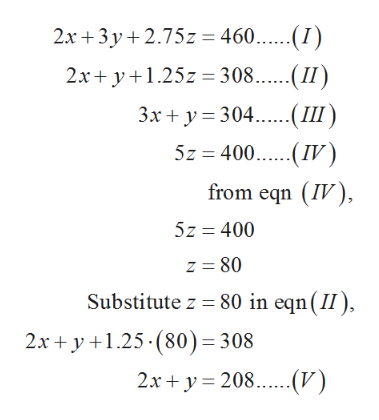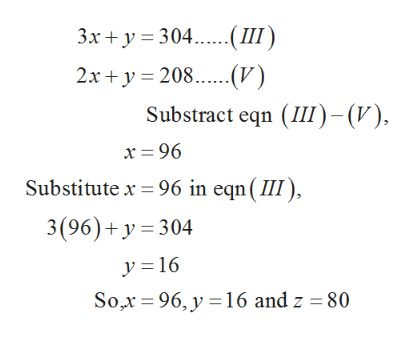# Assume that each gallon of Deluxe Vanilla uses 2 quarts of milk, 2 quarts of cream, and 3 ounces of vanilla; each gallon of Regular Vanilla uses 3 quarts of milk, 1 quart(s) of cream, and 1 ounces of vanilla; and each gallon of Deluxe Chocolate uses 2.75 quarts of milk, 1.25 quarts of cream, and 5 ounces of cacao. Assume also that the shop has on hand 115 gallons of milk, 77 gallons of cream, 19 pounds of vanilla, and 25 pounds of cacao. How many gallons of each type of ice cream should the shop make to use up all of these ingredients?When you setup a system of linear equations to solve this problem, how many variables and how many equations should you have.?Number of variables:  Number of equations:

Question
6 views

Assume that each gallon of Deluxe Vanilla uses 2 quarts of milk, 2 quarts of cream, and 3 ounces of vanilla; each gallon of Regular Vanilla uses 3 quarts of milk, 1 quart(s) of cream, and 1 ounces of vanilla; and each gallon of Deluxe Chocolate uses 2.75 quarts of milk, 1.25 quarts of cream, and 5 ounces of cacao. Assume also that the shop has on hand 115 gallons of milk, 77 gallons of cream, 19 pounds of vanilla, and 25 pounds of cacao. How many gallons of each type of ice cream should the shop make to use up all of these ingredients?

When you setup a system of linear equations to solve this problem, how many variables and how many equations should you have.?

Number of variables:

Number of equations:

check_circle

Step 1

Let x = Amount of deluxe vanilla to be produced

y = Amount of regular vanilla to be produced

z = Amount of deluxe chocolate to be produced

Since 1 gallon = 4 quarts

So, 115 gallons = 4x115 = 460 quarts

And, 77 gallons = 4x77 = 308 quarts

Since, 1 pound = 16 ounces

So, 19 pounds = 19x16 = 304 ounces

And, 25 pounds = 25x16 = 400 ounces

Now, according to the question the system of linear equations are written as,

Step 2

Solve the above system of the linear equation,help_outlineImage Transcriptionclose2x 3y 7= 460.....(I) 2x+y1 308..II 3x 304(III 5z 400(IV) from eqn (IV) 5z 400 z 80 Substitute z 80 in eqn (II) 2 x+y1.25-(80)= 308 2x 208(V) fullscreen
Step 3

From equation (III) and (V) find the value of...help_outlineImage Transcriptionclose3x y 304III 2x 208(V Substract eqn (III)-() Substitute x 96 in eqn(II) 3(96)+ y 304 y 16 Sox 96, y 16 and z = 80 fullscreen

### Want to see the full answer?

See Solution

#### Want to see this answer and more?

Solutions are written by subject experts who are available 24/7. Questions are typically answered within 1 hour.*

See Solution
*Response times may vary by subject and question.
Tagged in

### Math